## Not all charts need to be pretty!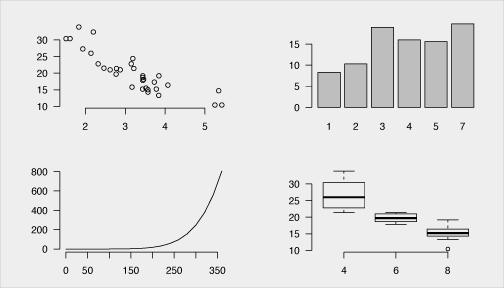## What to Remember from this Section

Exploratory data analysis plotting should be quick and simple and base R excels at this

Visualization Function
Strip chart `stripchart()`
Histogram `hist()`
Density plot `plot(density())`
Box plot `boxplot()`
Bar chart `barplot()`
Dot plot `dotchart()`
Scatter plot `plot()`, `pairs()`
Line chart `plot()`

## What to Remember from this Section

In R, graphs are typically created interactively:

```attach(mtcars)
plot(wt, mpg)
abline(lm(mpg~wt))
title("Regression of MPG on Weight")```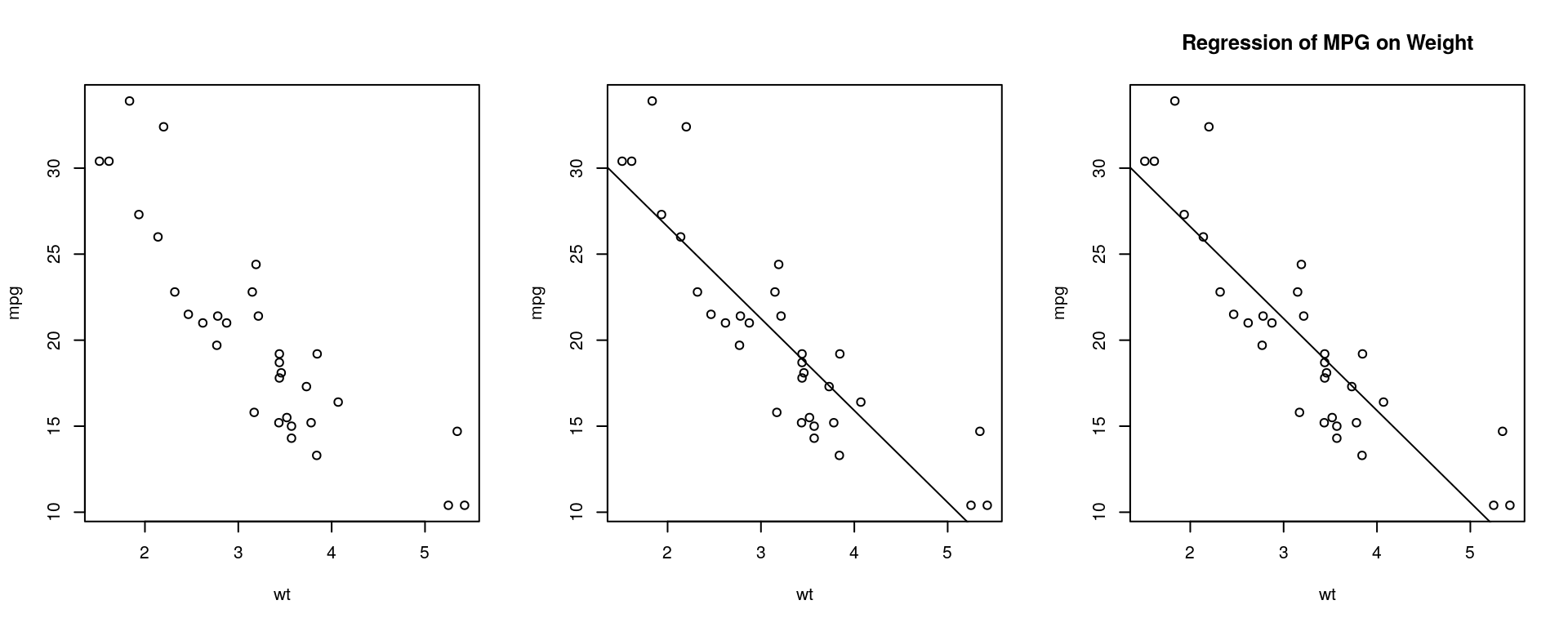## What to Remember from this Section

You can specify fonts, colors, line styles, axes, reference lines, etc. by specifying graphical parameters

This allows a wide degree of customization; however…

I have found that `ggplot` is an easier syntax for customization needs

## Data Used…

Import the following data sets from the data folder

```facebook.tsv
reddit.csv
race-comparison.csv
Supermarket-Transactions.xlsx```

## Continuous Variables: Strip Chart

Useful when sample sizes are small but not when sample size are large

```stripchart(mtcars\$mpg, pch = 16)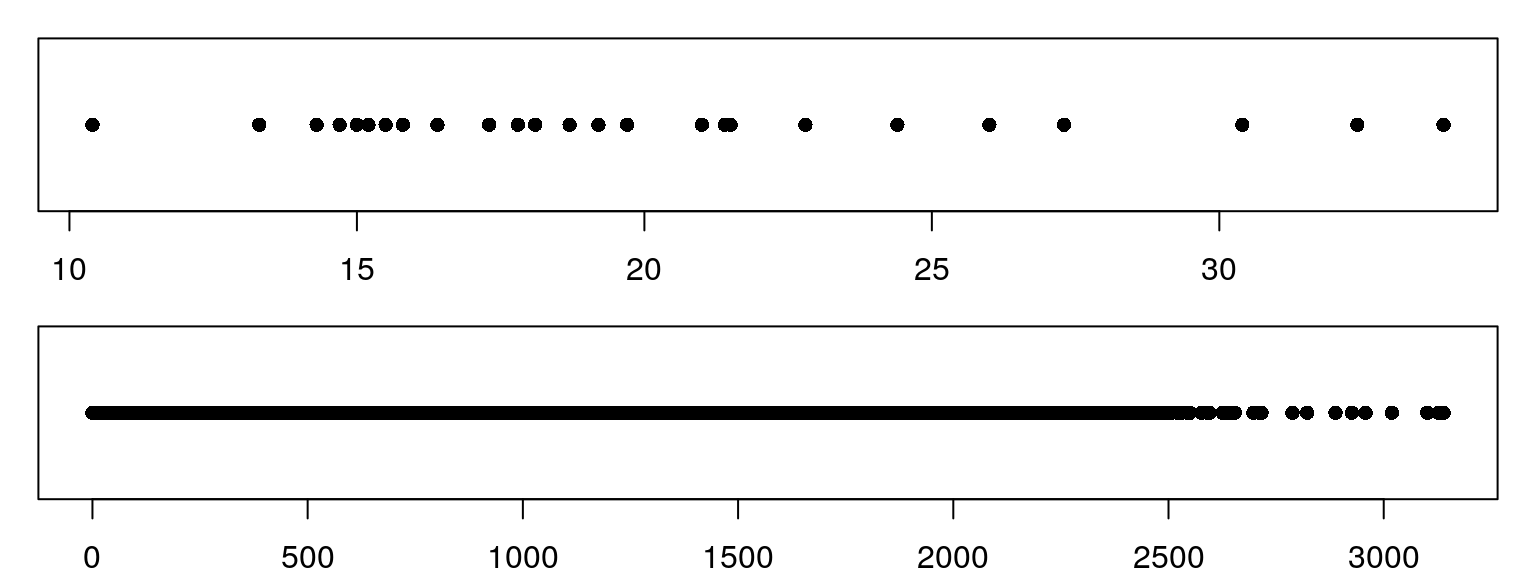## Continuous Variables: Histogram

```hist(facebook\$tenure)

hist(facebook\$tenure, breaks = 100, col = "grey", main = "Facebook User Tenure", xlab = "Tenure (Days)")```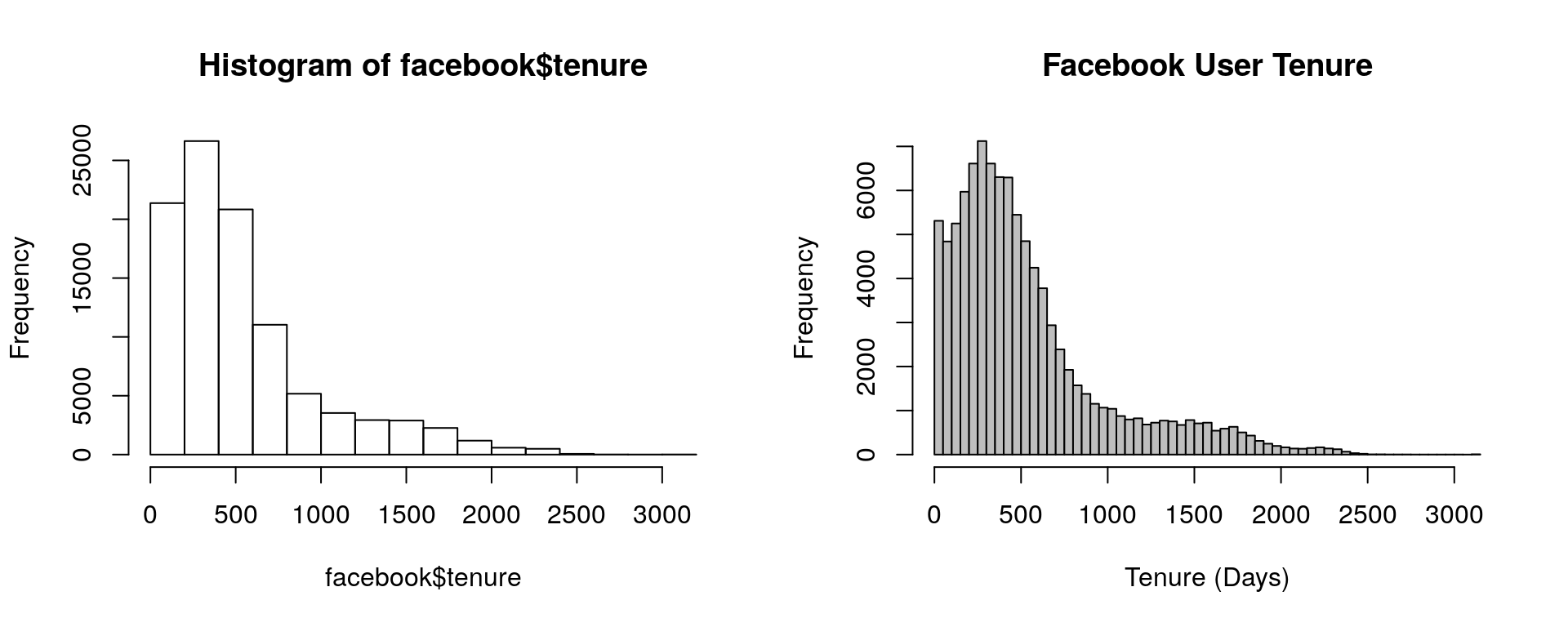## Continuous Variables: Histogram

A perfect example of why customization with base R is not always enjoyable; in ggplot this is far simpler

```x <- na.omit(facebook\$tenure)

# histogram
h<-hist(x, breaks = 100, col = "grey", main = "Facebook User Tenure", xlab = "Tenure (Days)")

xfit <- seq(min(x), max(x), length = 40)
yfit <- dnorm(xfit, mean = mean(x), sd = sd(x))
yfit <- yfit * diff(h\$mids[1:2]) * length(x)
lines(xfit, yfit, col = "red", lwd = 2)```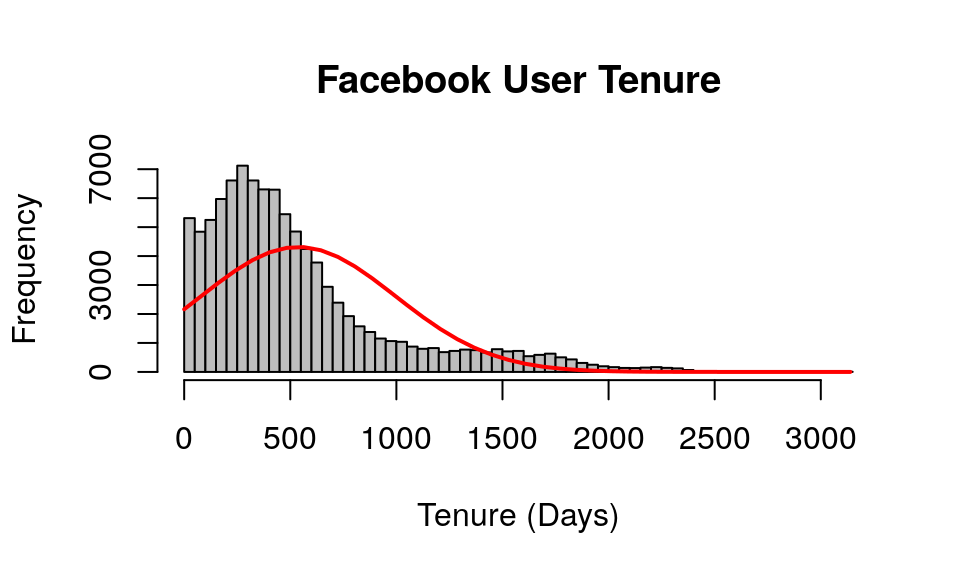## Continuous Variables: Density Plot

Enclose density(x) within plot()

```# basic density plot
d <- density(facebook\$tenure, na.rm = TRUE)

plot(d, main = "Kernel Density of Tenure")

# fill denisty plot by adding polygon()
polygon(d, col = "red", border = "blue")```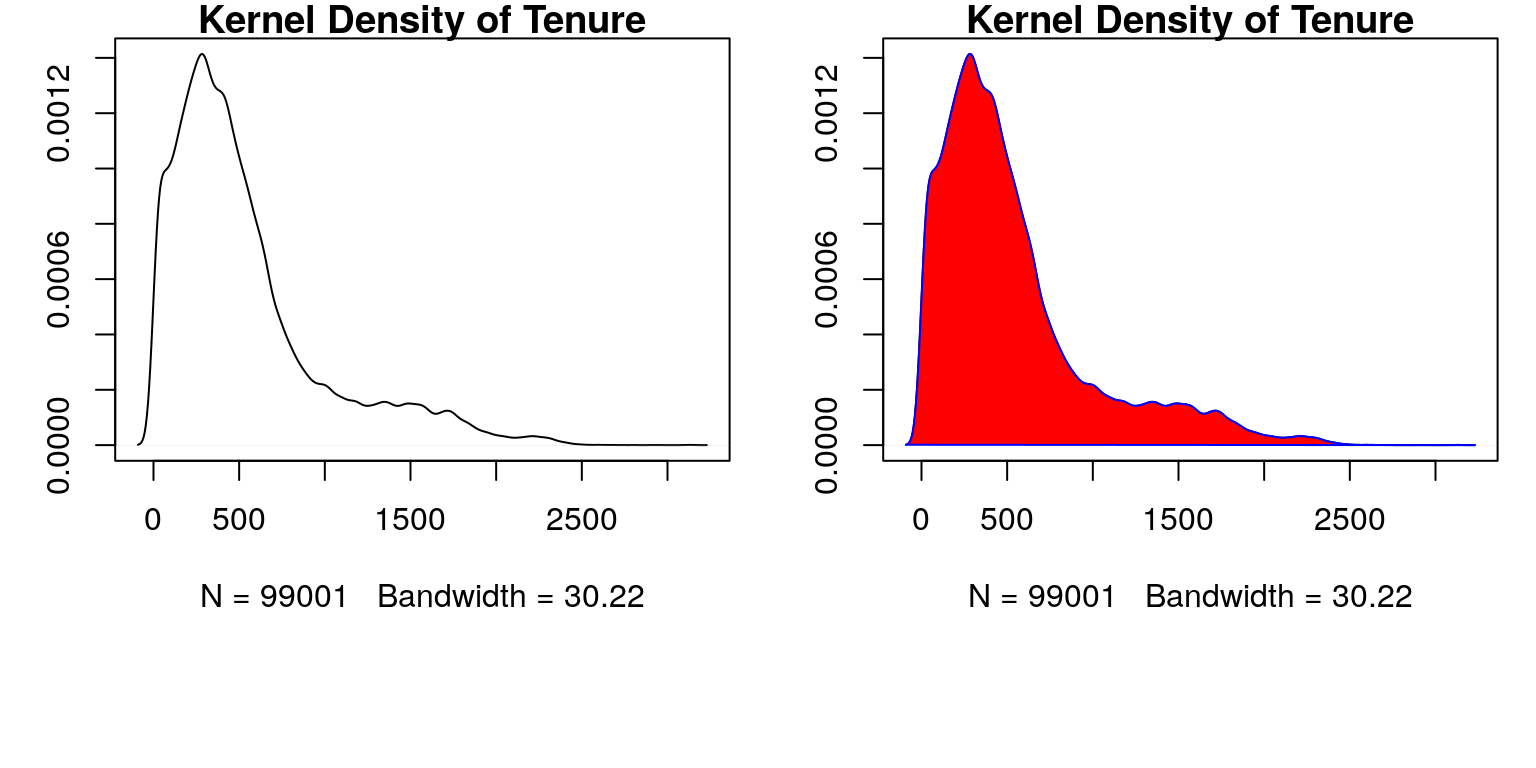## Continuous Variables: Box Plot

The previous methods provide good insights into the shape of the distribution but don't necessarily tell us about specific summary statistics such as:

`summary(facebook\$tenure)`
```##    Min. 1st Qu.  Median    Mean 3rd Qu.    Max.    NA's
##     0.0   226.0   412.0   537.9   675.0  3139.0       2```

However, boxplots provide a concise way to illustrate these standard statistics, the shape, and outliers of data: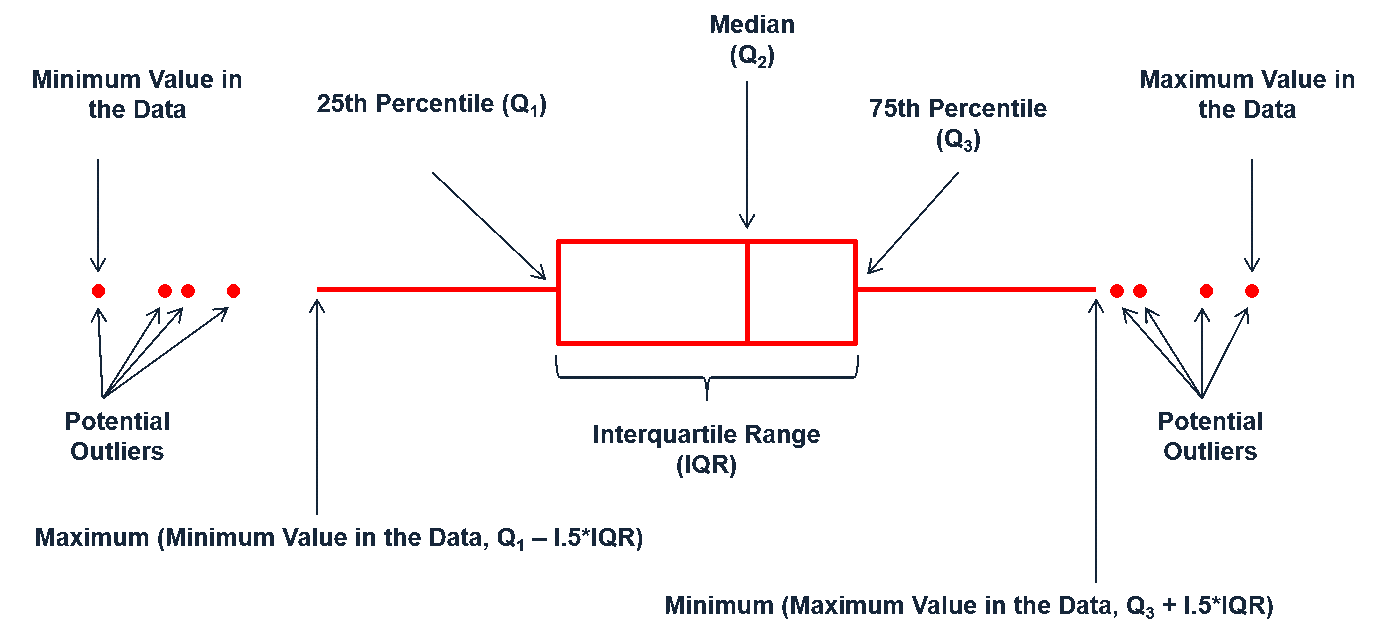## Continuous Variables: Box Plot

```boxplot(facebook\$tenure, horizontal = TRUE)
boxplot(facebook\$tenure, horizontal = TRUE, notch = TRUE, col = "grey40")```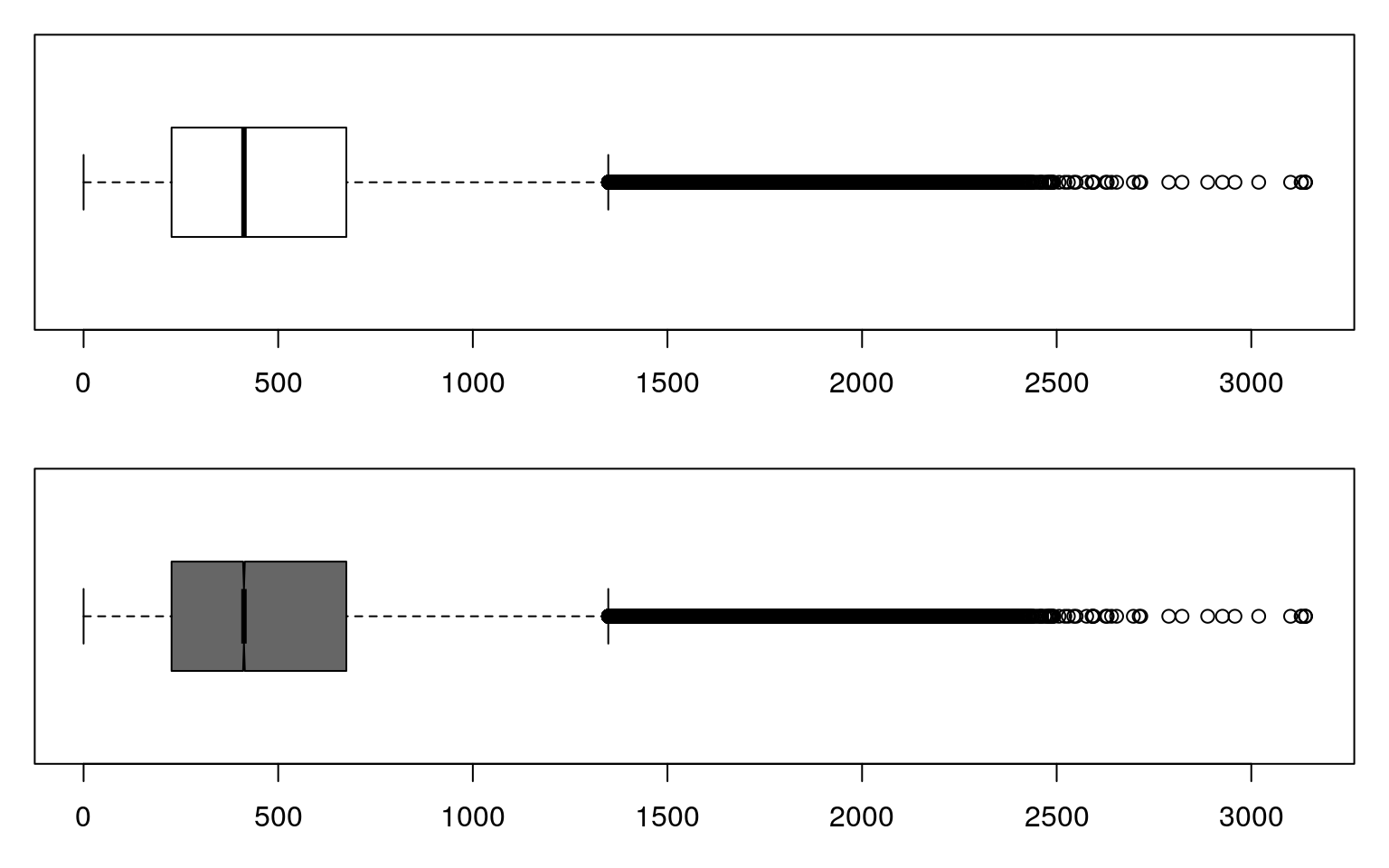Using the `facebook.tsv` data…

Visually assess the continuous variables. What do you find?

## Categorical Variables: Bar Chart

```reddit <- read.csv("../data/reddit.csv")

table(reddit\$dog.cat)
##
##    I like cats.    I like dogs. I like turtles.
##           11156           17151            4442

barplot(table(reddit\$dog.cat))```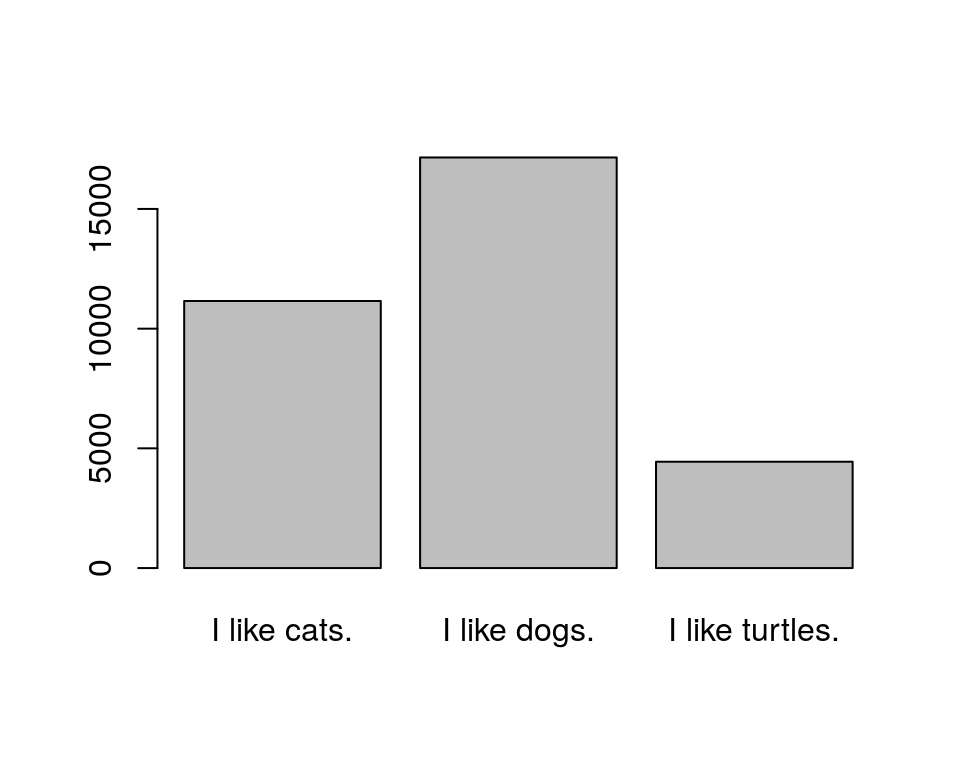## Categorical Variables: Bar Chart

```pets <- table(reddit\$dog.cat)

barplot(pets, main = "Reddit User Animal Preferences", col = "cyan")

par(las = 1)
barplot(pets, main = "Reddit User Animal Preferences", horiz = TRUE, names.arg = c("Cats", "Dogs", "Turtles"))```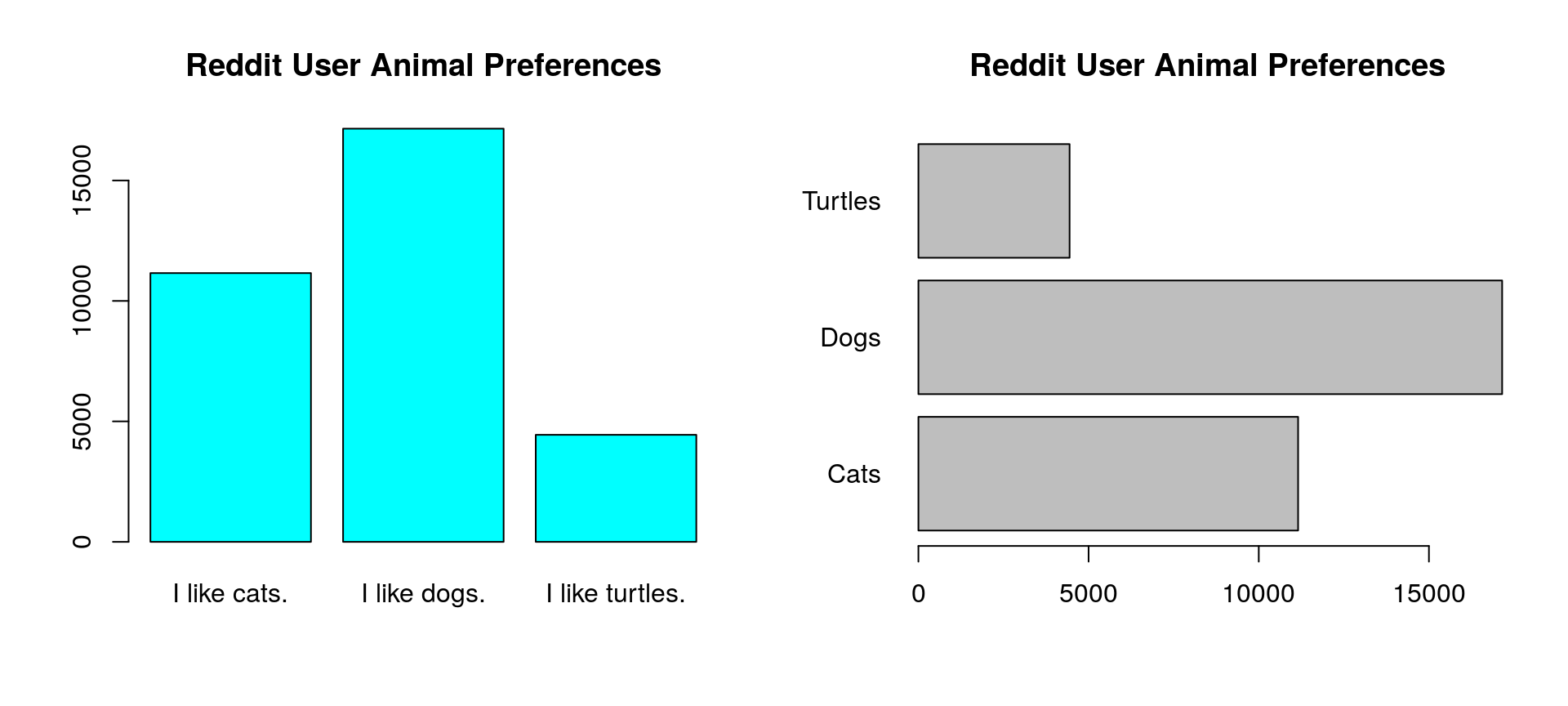## Categorical Variables: Bar Chart

What if we want to visualize data regarding many categories…

```library(dplyr)

state <- reddit %>%
group_by(state) %>%
tally() %>%
arrange(n) %>%
filter(state != "")

state```
```## # A tibble: 52 x 2
##            state     n
##           <fctr> <int>
##  1       Ontario     1
##  2       Wyoming    20
##  3  South Dakota    28
##  4  North Dakota    34
##  5       Montana    46
##  6   Mississippi    48
##  7 West Virginia    51
##  8      Delaware    59
##  9        Hawaii    68
## 10  Rhode Island    72
## # ... with 42 more rows```

## Categorical Variables: Bar Chart

Bar charts work but…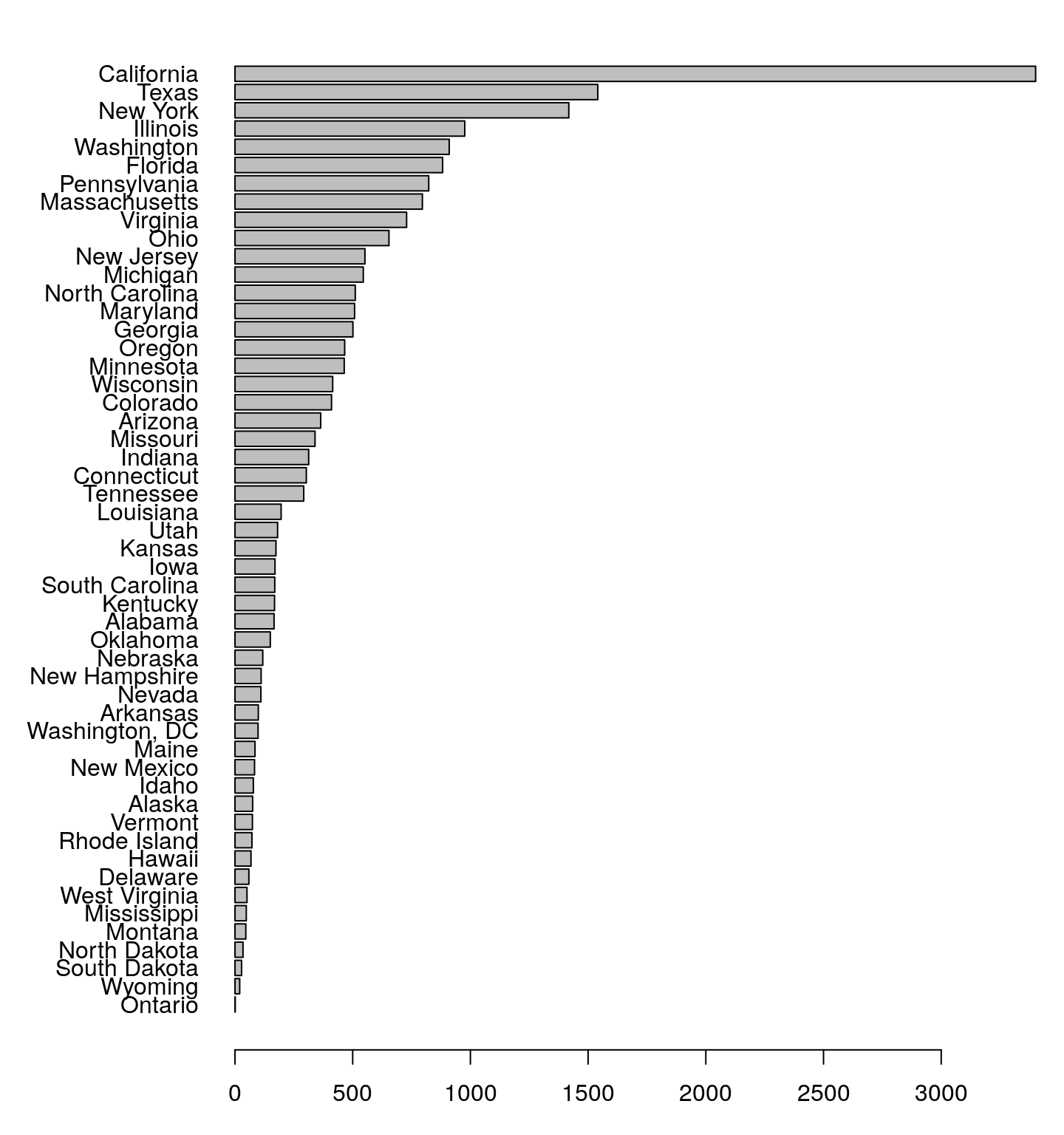## Categorical Variables: Dot Plot

dot plots provide less noise

`dotchart(state\$n,labels = state\$state, cex = .7)`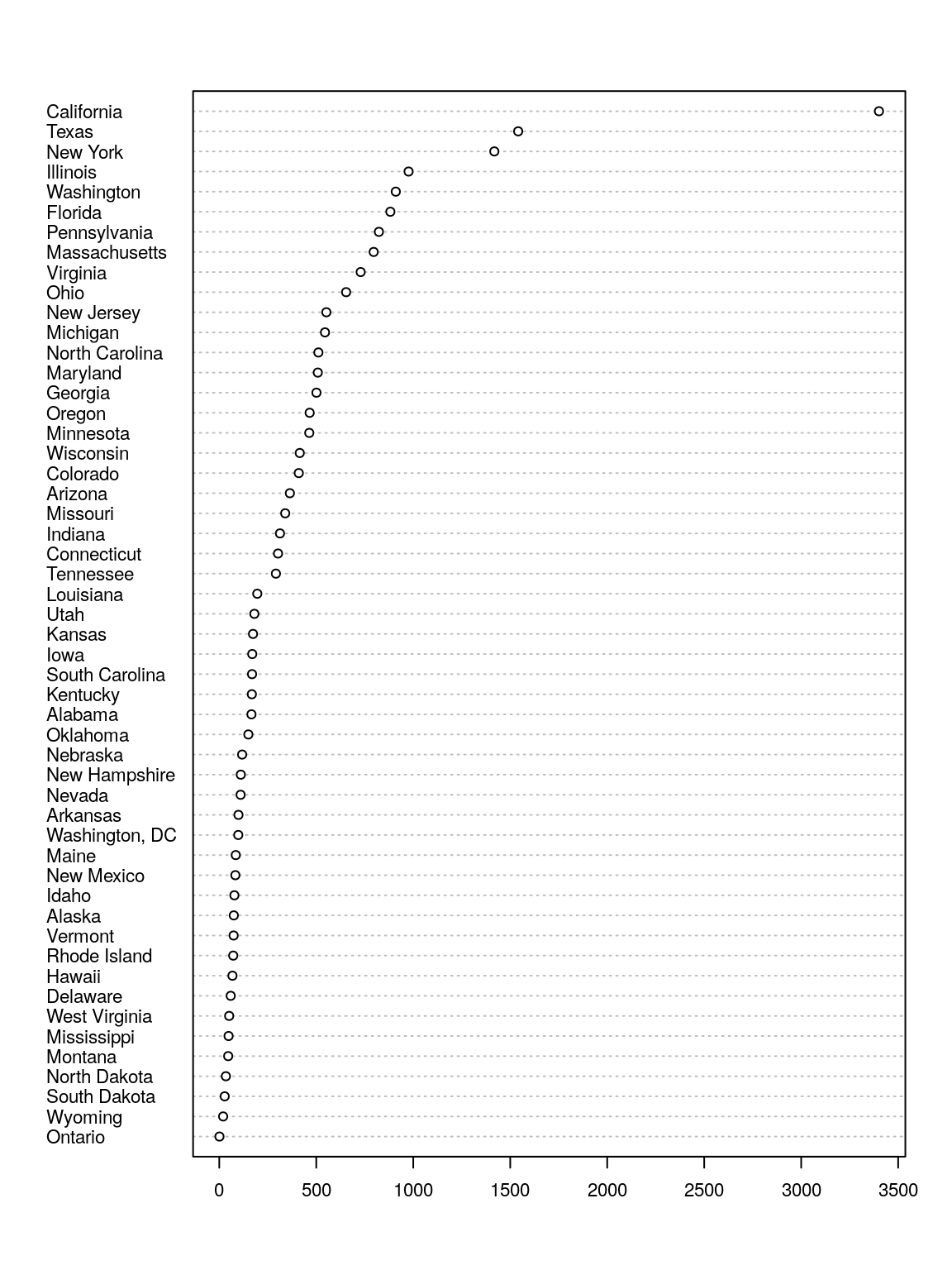Using the `reddit.csv` data…

1. Assess the frequency of education levels. What does this tell you?

Hint: preceed your plot function with `par(mar = c(5,15,1,1), las = 2)`

2. Assess how the different cheeses rank with Reddit users. What does this tell you?

## Scatter Plot

```plot(x = race\$White_unemployment, y = race\$Black_unemployment, pch = 16, col = "blue")
plot(x = race\$Black_unemployment, y = race\$black_college, pch = 16, col = "blue")```